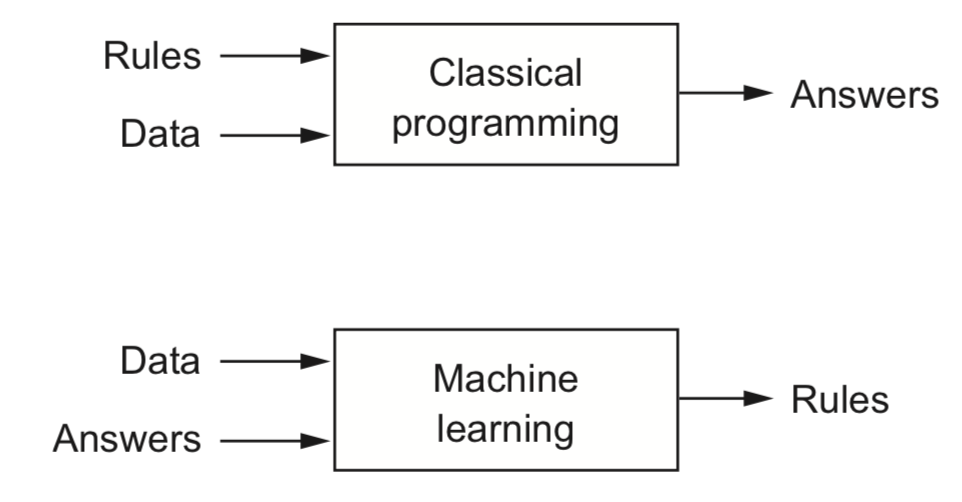# A Brief Introduction To Artificial Intelligence

Let’s start with the first question people asked themselves then as they do now: Are computers able to think?

This gave rise to the first ideas and visions of A.I. as a predefined set of rules. In the beginning, A.I. had nothing to do with deep learning or machine learning. Therefore it can range from simple to extremely complex today.

Let’s take a closer look at these two models. We take a look at machine learning as our main field of interest and have a short glance at neural networks, which are a part of machine learning.

## 1. Machine Learning

The first sub-category of A.I. is machine learning.

Its job is to discover the rules for us based on the data. We define the data that is fed in to our model and determine our final goal. As you probably can imagine, this technique can lead to inaccuracies. This can be a problem as the goal of machine learning is to achieve the highest possible accuracy.### 1.1 Supervised Learning

With this type of machine learning, we already know beforehand which product we want to receive in the end. Therefore, we can compare it to the desired end result at every step.

The expectations are compared again and again with the desired result and the algorithm adjusted until we are satisfied with the accuracy or the result.### 1.2 Unsupervised Learning

Here we have the exact difference. We don’t have the final result yet. Basically we try to find out how the data is related and try to divide it into groups.

Like this we are able to determine what end result we want to reach.### 1.3 Reinforcement Learning

This time there is no direct data. This system is based on an agent, the environment and a reward system. These rewards can be positive or negative.

The agent’s goal is to collect as many “reward points” as possible. Just imagine a child learning to read and everytime it gives us a correct answer it will get a cookie.## 2. Neural Networks

A neural network is a subclass of machine learning. This uses a layered representation of data.

In the last paragraph we spoke about two layers of complexity. The data fed in and the data output. However, a neural network can have several layers that transform or process data in a wide variety of ways.These additional layers are also called “hidden layers”. They are adjusted by means of rules and weightings (even several times) until the data is output again.

I hope this short introduction gave you a basic understanding about A.I. and you understood that A.I. can be a automated mouse click as well as natural language understandig chatbot or a NPC in a game. Maybe think about situation where you could you the different models.